upm  1.7.1 Sensor/Actuator repository for libmraa (v2.0.0)
ABP Class Reference

API for the ABP Honeywell Pressure Sensor. More...

## Detailed Description

This is the UPM Module for the ABP Honeywell Pressure and Temp sensor. This sensor uses an onboard ASIC to output values of pressure that are updated at approximately 2 KHz. It is capable of detecting pressure in the 0-5 psi range and it has an i2c based interface. Temperature calculation using this driver is possible but not all ABP sensors support that functionality.

NOTE: This driver supports only the I2C based ABP sensors, it does not currently support SPI and Analog based sensors.// Instantiate an ABP sensor on i2c bus 0
while (shouldRun) {
abp.update();
cout << "Retrieved pressure: " << abp.getPressure() << endl;
cout << "Retrieved Temperature: " << abp.getTemperature() << endl;
upm_delay(1);
}

## Public Member Functions

ABP (int bus, int devAddress)

~ABP ()

float getPressure ()

float getTemperature ()

void update ()

void setMaxPressure (int max)

void setMinPressure (int min)

## Constructor & Destructor Documentation

 ABP ( int bus, int devAddress )

ABP constructor

Parameters
 bus i2c bus to be used devAddress i2c address of the sensor
 ~ABP ( )

ABP destructor

Here is the call graph for this function: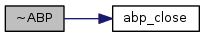## Member Function Documentation

 float getPressure ( )

Function to get the compensated pressure. The datasheet provides a way to calculate the pressure. In order to know the units take a look at the datasheet.

Returns
float compensated temperature value

Here is the call graph for this function: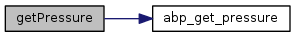float getTemperature ( )

Function to retrieve the compensated temperature values. All ABP seem to calculate temperature in degree Celsius. (The above fact needs to be verified) NOTE: This functionality is not available in all the ABP sensors.

Returns
float compensated temperature value

Here is the call graph for this function: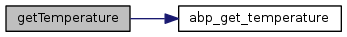void update ( )

This functio has to be called before calling either of the get temperature or get pressure functions. This function updates the device context with the latest values. Not calling this function will lead to stale values.

Here is the call graph for this function: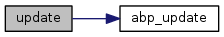void setMaxPressure ( int max )

Function to set the max pressure that the sensor can read, can be retrieved from the datasheet, if this function is not explicitly called to the set the min value, then a default max value of 5 will be set.

Parameters
 max int maximum pressure value possible

Here is the call graph for this function: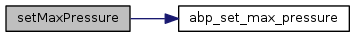void setMinPressure ( int min )

Function to set the min pressure that the sensor can read, can be retrieved from the datasheet, if this function is not explicitly called to the set the min value, then a default min value of 0 will be set.

Parameters
 min int minimum pressure value possible

Here is the call graph for this function: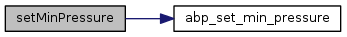The documentation for this class was generated from the following files: